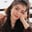Related Tags

python

# What is a pandas autocorrelation plot in Python?Fouzia Bashir

## Overview

Autocorrelation plots are specifically used to check the randomness between data points of a dataset. In time series analysis, correlation is computed parallel to each data point at varying time lags to determine their relationship.

pandas.plotting.autocorrelation_plot()

### Syntax

pandas.plotting.autocorrelation_plot(series, ax=None, **kwargs)

### Parameters

• series: It should be a time series instance.
• ax: It shows matplotlib axis object. Its default value is None.
• **kwargs: These are keyword arguments.

### Return value

It returns a matplotlib.axis.Axes object.

### Example

In this example, we draw an autocorrelation plot on randomly generated data points.

# importing libraries
import pandas as pd
import matplotlib.pyplot as plot
import numpy as np
# creating a sample space of 500 values
data = np.linspace(-10, np.pi*5, num=500)
# creating a series of random values
_series = pd.Series(np.cos(data) * np.random.rand(500))
# generate autocorrelation plot
pd.plotting.autocorrelation_plot(_series)
# save above generated graph as PNG file in output directory
plot.savefig("output/graph.png")

### Explanation

• Lines 2–4: We load the pandas, matplotlib, and numpy libraries.
• Line 6: We invoke np.linspace() to generate 500 sample values between -10 and np.pi * 5.
• Line 8: We generate a _series series of random numbers.
• Line 10: The pd.plotting.autocorrelation_plot() method generates an autocorrelation plot of the above-created series of random numbers.
• Line 12: We save the graph in the output directory as a PNGPortable Network Graphics file.

RELATED TAGS

python

CONTRIBUTORFouzia Bashir
RELATED COURSES

View all Courses

Keep Exploring

Learn in-demand tech skills in half the time